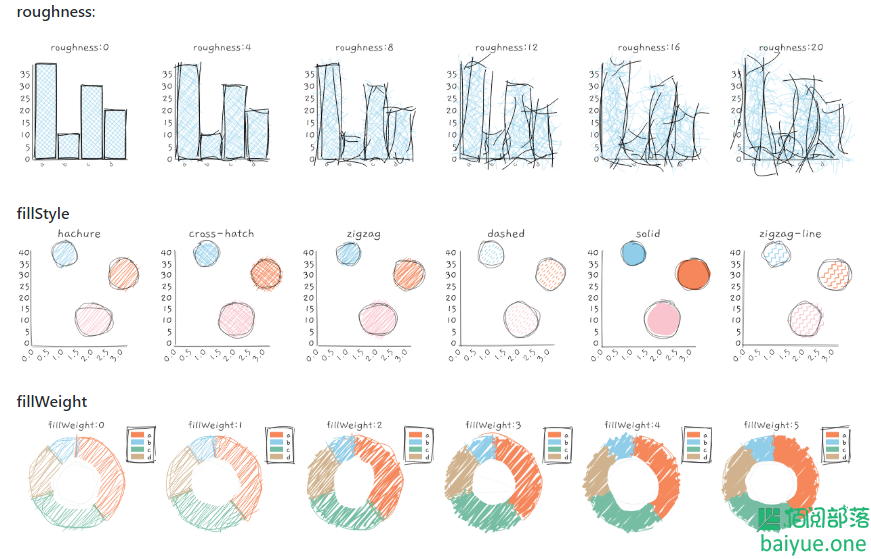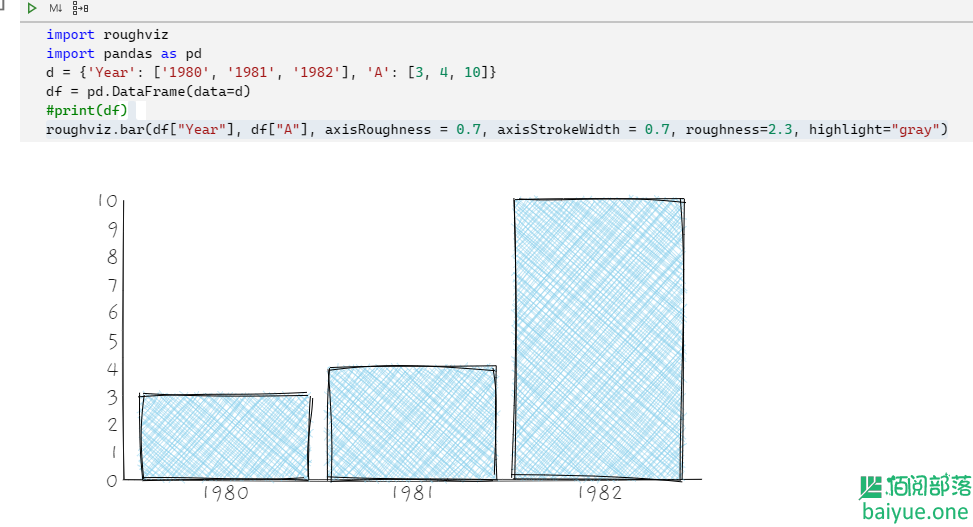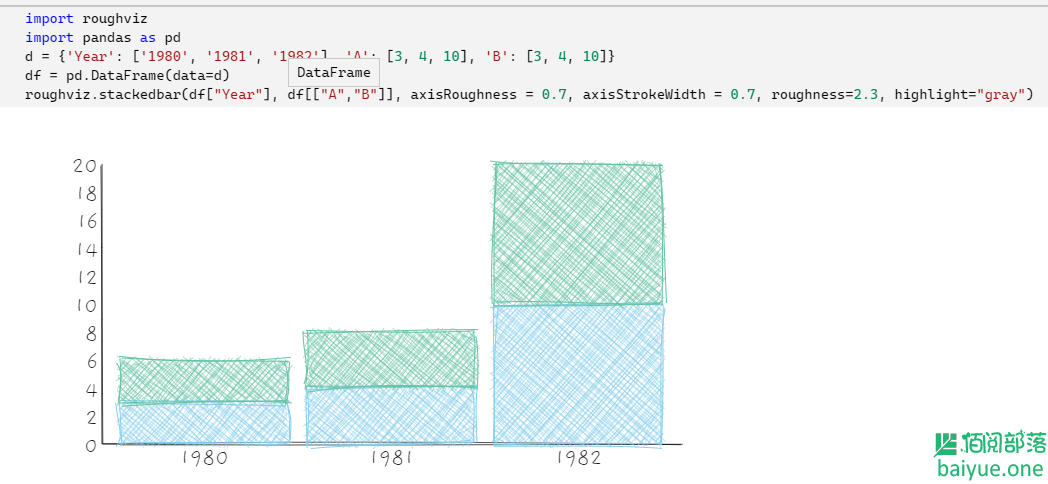• 欢迎访问佰阅部落
• 如果您觉得本站非常有看点，那么赶紧使用Ctrl+D 收藏吧

# roughViz：目前见过最好的手绘风格图表绘制工具

3年前 (2020-07-09) 3199次浏览

`oughViz.js`是一款非常有趣的手绘风格图表绘制工具，不仅支持CDN引入js标签的方式，还支持`vue``python``react`。支持几乎所有常见的图表类型，如果你大胆使用这种风格，必将收获不少惊喜。下面就来具体介绍下。## 0. 关于安装

### CDN模式：浏览器直接引入

``````<script src="https://unpkg.com/rough-viz@1.0.6"></script>
``````

### VUE模式：

``````npm install vue-roughviz
``````

### Python模式：

``````pip install roughviz
#或者
pip install py-roughviz
``````

## 1. 使用演示：以python为例

``````import roughviz
import pandas as pd
d = {'Year': ['1980', '1981', '1982'], 'A': [3, 4, 10]}
df = pd.DataFrame(data=d)
print(df)
roughviz.bar(df["Year"], df["A"], axisRoughness = 0.7, axisStrokeWidth = 0.7, roughness=2.3, highlight="gray")
````````````import roughviz
import pandas as pd
d = {'Year': ['1980', '1981', '1982'], 'A': [3, 4, 10], 'B': [3, 4, 10]}
df = pd.DataFrame(data=d)
roughviz.stackedbar(df["Year"], df[["A","B"]], axisRoughness = 0.7, axisStrokeWidth = 0.7, roughness=2.3, highlight="gray")
``````## 2. 使用演示：以WEB浏览器为例